# A Brief Introduction To Romberg Integration

This blog briefly describes a remarkable integration algorithm, called "Romberg integration." The algorithm is used in the field of numerical analysis but it's not so well-known in the world of DSP.

To show the power of Romberg integration, and to convince you to continue reading, consider the notion of estimating the area under the continuous x(t) = sin(t) curve based on the five x(n) samples represented by the dots in Figure 1.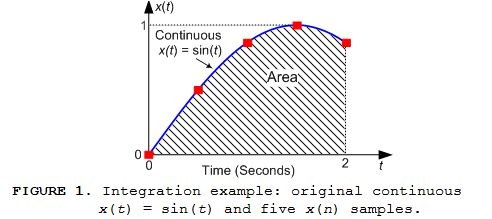The results of performing a Trapezoidal Rule, a Simpson's 1/3 Rule, and a Romberg integration using Figure 1's five x(n) samples are given in Table 1. There we see the profound reduction in integration error afforded by Romberg integration.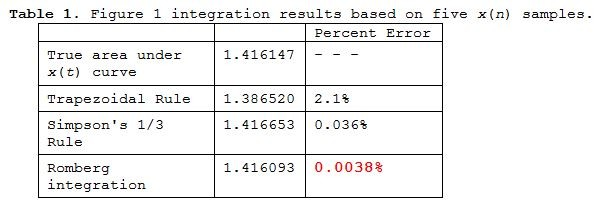To achieve Table 1's low 0.0038% Romberg error a Trapezoidal Rule integration would require 100 x(n) samples rather than just the five x(n) samples in Figure 1. To learn more about Romberg integration please read on.

Romberg Integration

Romberg integration is a process used to compute finite integrals requiring very high accuracy (super‑low error). This integration process, first proposed by Werner Romberg in 1955, is remarkable because it employs a series smaller‑sized less‑accurate integral approximations, typically obtained using the Trapezoidal Rule integration method, to compute a much more accurate final definite integral approximation [1-3].

A simplified depiction of the Romberg integration process used to compute the Romberg integration values in the bottom row of Table 1 is shown in Figure 2.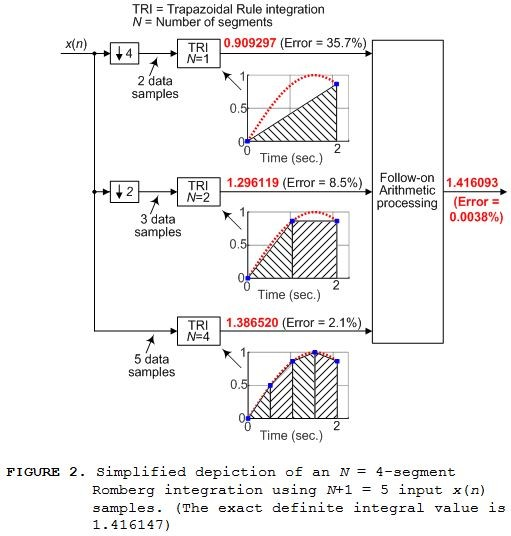The 'TRI' notation in Figure 2 means Trapezoidal Rule integration and the ↓k symbol represents downsampling by integer k. We see in Figure 2 that separate four-segment, three-segment, and two-segment Trapezoidal Rule integrations are performed.

Figure 2 is called an "N = 4-segment Romberg integration" because five input x(n) samples enables the total integrated area to be divided into N = 4 smaller area segments as shown by the bottom integration in Figure 2. As it turns out, log2(N)+1 individual trapezoidal integration operations are required for a single N-segment Romberg integration process.

The full block diagram of the N = 4-segment Romberg integration is given in Figure 3.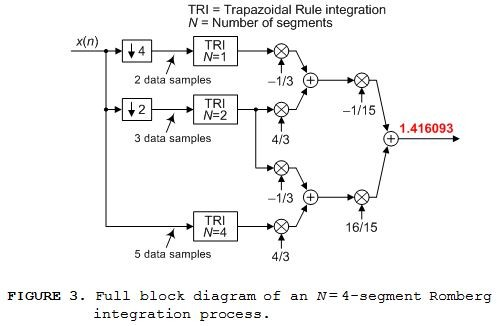If we had nine x(n) signal samples of Figure 1's continuous x(t) signal, between t = 0 and t = 2 seconds, we could perform the N = 8‑segment Romberg integration shown in Figure 4. In that case our computed integral value is 1.4161469 having a percent error of 6x10‑6%.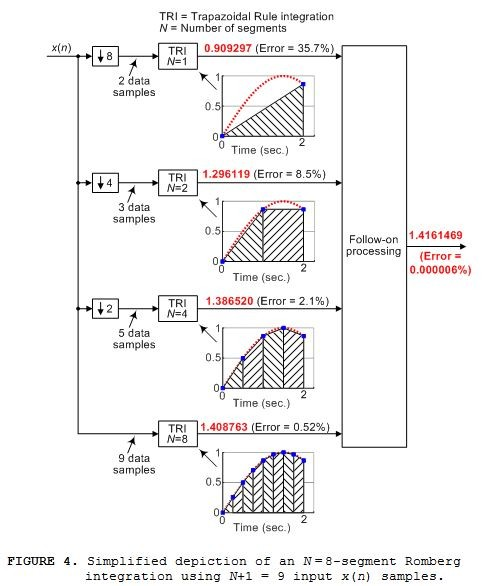The full block diagram of the N = 8-segment Romberg integration is given in Figure 5.An N = 16 Integration Example

Below I present the N = 16‑segment (17 signal samples) estimated definite integral of the continuous x(t), over the time range of 0 ≤ t ≤ p, defined by: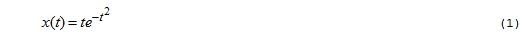shown in Figure 6.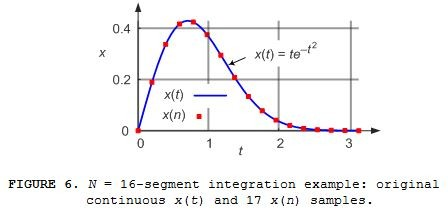The Figure 6 estimated integral results are given in Table 2. There we see the improved accuracy, for this particular x(n) sequence, of our Romberg integration. To achieve Table 2's 3.6x10-4% Romberg error a Trapezoidal Rule integration would require 680 x(n) samples rather than just the 16 x(n) samples in Figure 6.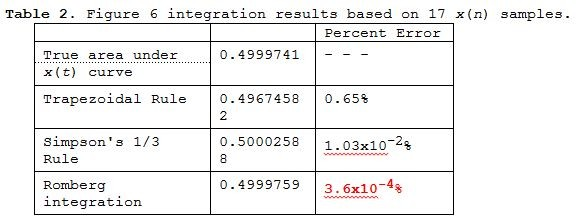To keep this blog from being too lengthy I conclude my discussion here. If Romberg integration interests you, the following additional information

• Further details, and references, regarding Romberg integration
• How to compute the multiplication coefficients in Figures 3 and 5
•  MATLAB code implementing Romberg integration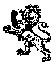[ - ]
Comment by February 24, 2019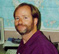Very interesting article Rick... Thanks!

[ - ]
Comment by January 23, 2019Neat! I'll have to add to my mental toolbox.

The DSP applications may be not-well known because they are limited; use of Romberg integration makes sense for analytical functions whose derivatives are continuous, but for numerical data containing noise, the higher-order integrator methods aren't going to add any accuracy compared to a trapezoidal integration.

[ - ]
Comment by January 23, 2019Hi jms_nh.

You are correct. Using 8-segment or 16-segment Romberg integration is useful so long as the input signal's derivative is continuous and only changes polarity once. Romberg integration is not particularly effective for input waveforms like the following:If I had to integrate the above waveform I'd explore the idea of breaking the above waveform into three separate (smaller) waveforms where each smaller waveform contained no discontinuity.

jms_nh, I confess, I was lazy! I did not study/compare the performance of Trapezoidal Rule, Simpson's 1/3 Rule, or Romberg integration when the input signal was contaminated with noise.

[ - ]
Comment by February 25, 2019I ran a quick script to test the noise idea out.  For very low noise, Romberg works better, but there's a crossover point of performance, where the TRI method works better than Romberg.  For the example of a sinewave from t= 0 -> 2, with 5 points, it was about sigma=0.07.

[ - ]
Comment by February 1, 2019Excellent article, Rick

A Romberg Integration function is also available in the R programming language.

[ - ]
Comment by February 1, 2019Hi woodpecker.

Thanks for the R language link!

[ - ]
Comment by February 25, 2019It looks like Romberg integration uses Richardson Extrapolation, which can also be used to estimate rate of convergence when the true value isn't known a priori.  Running through that would make a great follow up blog post!

[ - ]
Comment by March 1, 2019Thanks Rick,

I found something similar of interest which uses interpolation algorithm based on the Sampling theorem

INTEGRATION OF SAMPLED SIGNALS S J C Dyne and J K Hammond

[ - ]
Comment by March 1, 2019Hi kvengatt. Thanks for the link. Unfortunately I don't qualify to view that "integration" paper.

[ - ]
Comment by March 5, 2019Am not sure if i can upload this paper as its copyrights are owned by IEEE. Is there a clean way of sharing it ? Kindly let me know

[ - ]
Comment by March 5, 2019Hi kvengatt. You're being honorable. Good for you. I used to be an Associate Editor for the IEEE Signal Processing Magazine and as far as I know only the authors of a published paper are permitted to distribute copies of their published paper.

To post reply to a comment, click on the 'reply' button attached to each comment. To post a new comment (not a reply to a comment) check out the 'Write a Comment' tab at the top of the comments.